Courses

# Polynomials - Practice Test

## 10 Questions MCQ Test Quantitative Aptitude for Banking Preparation | Polynomials - Practice Test

Description
This mock test of Polynomials - Practice Test for UPSC helps you for every UPSC entrance exam. This contains 10 Multiple Choice Questions for UPSC Polynomials - Practice Test (mcq) to study with solutions a complete question bank. The solved questions answers in this Polynomials - Practice Test quiz give you a good mix of easy questions and tough questions. UPSC students definitely take this Polynomials - Practice Test exercise for a better result in the exam. You can find other Polynomials - Practice Test extra questions, long questions & short questions for UPSC on EduRev as well by searching above.
QUESTION: 1

Solution:

(2)

QUESTION: 2

Solution:

Ans : (1)

QUESTION: 3

### Find a quadratic polynomial, the sum and product of whose zeroes are -7 and -2

Solution:

Ans : (3)

QUESTION: 4

Find a cubic polynomial when the zeroes are 3, -1,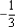Solution:

1

QUESTION: 5

Find a quadratic polynomial, the sum and product of whose zeroes are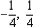Solution:
QUESTION: 6

Find all the zeroes of 2x4 - 3x3 - 3x2 + 6x - 2 if two of its zeroes are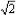and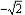Solution:
QUESTION: 7

Find the quotient and remainder when the polynomial x4 - 3x2 + 4x + 5 is divided by x2 - x + 1

Solution: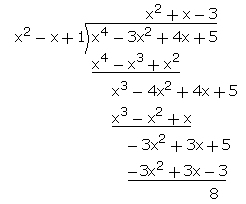Quotient = x^2 + x - 3

Remainder = 8

QUESTION: 8

The quotient and remainder are x - 2 and -2x + 4 respectively. If the polynomial x3 - 3x2 + x + 2 is divided by g(x). Find g(x)

Solution:
QUESTION: 9

Find P and q, if the zeroes of the polynomial x3 - 3x2 + x + 1 are p-q, p, p + q

Solution:
QUESTION: 10

Find a quadratic polynomial whose zeroes are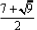and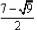Solution: